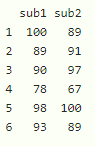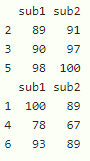Open in App
Not now

# Select Rows if Value in One Column is Smaller Than in Another in R Dataframe

• Last Updated : 23 Sep, 2021

In this article, we will discuss how to select rows if the value in one column is smaller than another in dataframe in R programming language.

Data frame in use:## Method 1: Using Square Brackets

By using < operator inside the square bracket we can return the required rows.

Syntax:

dataframe[dataframe\$column1 < dataframe\$column2,]

where,

• dataframe is the input dataframe
• column1 is the first column
• column2 is the second column

Example: R program to select rows only if first column values is less than second column values

## R

 `# create a dataframe with 6 rows and 2 columns ` `data=``data.frame``(sub1=``c``(100,89,90,78,98,93), ` `                ``sub2=``c``(89,91,97,67,100,89)) ` ` `  `# select rows only if first column values  ` `# is less than second column values ` `print``(data[data\$sub1 < data\$sub2,] ) ` ` `  `# select rows only if second column values  ` `# is less than first column values ` `print``(data[data\$sub2 < data\$sub1,] ) `

Output:## Method 2 : Using subset() function

This function gets the subset of the data from the dataframe where the condition is specified.

Syntax:

subset(dataframe, column1<column2)

where,

• dataframe is the input dataframe
• column1 is the first column in the dataframe
• column2 is the second column in the dataframe

Example: R program to select rows where first column is less than column2

## R

 `# create a dataframe with 6 rows and 2 columns ` `data=``data.frame``(sub1=``c``(100,89,90,78,98,93), ` `                ``sub2=``c``(89,91,97,67,100,89)) ` ` `  `# select rows only if first column values is less than  ` `# second column values using subset() function ` `print``(``subset``(data,sub1

Output:## Method 3 : Using filter() function

This function get the filtered data from the dataframe where the condition is specified. This is available in dplyr() package. So we need to install and load the package into the working space first.

Syntax:

filter(dataframe, column1<column2)

where,

• dataframe is the input dataframe
• column1 is the first column in the dataframe
• column2 is the second column in the dataframe

Example: R program to select rows where the first column is less than column2

## R

 `# load the package ` `library``(``"dplyr"``) ` ` `  `# create a dataframe with 6 rows and 2 columns ` `data=``data.frame``(sub1=``c``(100,89,90,78,98,93), ` `                ``sub2=``c``(89,91,97,67,100,89)) ` ` `  `# select rows only if first column values is less than  ` `# second column values using filter() function ` `print``(``filter``(data,sub1

Output:My Personal Notes arrow_drop_up
Related Articles